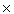Easysurf Home Site Map E-Mail Help Measurement Conversion Directory

# Base Number - Decimal Number Conversion

Decimal to Binary
Binary to Decimal
Binary Fraction to Decimal Fraction
Decimal to Ternary
Ternary to Decimal
Ternary Fraction to Decimal Fraction
Decimal to Quaternary
Quaternary to Decimal
Quaternary Fraction to Decimal Fraction
Decimal to Any Radix Base Number (between 2 and 36)
Any Radix Base Number (between 2 and 36) to Decimal
Convert a number in one base (between 2 and 36) to the equivalent number in another base (between 2 and 36)

Numerals Only
Numerals Only Directions
Decimal Number to Any Radix Base Number
Any Radix Base Number to Decimal
Convert numerals in one base (radix) to the equivalent numerals in another base - numerals only

## Convert Decimal to Binary (base 10 to base 2)

Enter Decimal Number: Binary

## Convert Binary to Decimal (base 2 to base 10)

Enter Binary Number: Decimal

## Convert Binary Fraction to Decimal Fraction (base 2 to base 10)

Enter Binary Fraction:
Decimal Fraction

## Convert Decimal to Ternary (base 10 to base 3)

Enter Decimal Number: Ternary

## Convert Ternary to Decimal (base 3 to base 10)

Enter Ternary Number: Decimal

## Convert Ternary Fraction to Decimal Fraction (base 3 to base 10)

Enter Ternary Fraction:
Decimal Fraction

## Convert Decimal to Quaternary (base 10 to base 4)

Enter Decimal Number: Quaternary

## Convert Quaternary to Decimal (base 4 to base 10)

Enter Quaternary Number: Decimal

## Convert Quaternary Fraction to Decimal Fraction (base 4 to base 10)

Enter Quaternary Fraction:
Decimal Fraction

## Convert Hexadecimal Fraction to Decimal Fraction (base 16 to base 10)

Decimal Fraction

## Convert Decimal to Any Radix Base Number (between 2 and 36)

Enter Base Number:
Enter Decimal Number:

## Convert Any Radix Base Number (between 2 and 36) to Decimal

Enter Base Number:
Enter a Number: Decimal

## Convert Any Radix Base Number (between 2 and 9) Fraction to Decimal Fraction

Enter Base Number (between 2 and 9):
Enter a Fraction:
Decimal

## Convert Any Radix Base Number (between 10 and 36) Fraction to Decimal Fraction

Enter Base Number (between 2 and 36):
Enter a Fraction:
Decimal

## Convert a number in one base (between 2 and 36) to the equivalent number in another base (between 2 and 36)

Enter from Base Number:
Enter from Number:
Enter to Base Number:

## Convert a Decimal Number to Any Radix Base Number numerals only

Enter Base Number:
Enter Decimal Number:

## Convert Any Radix Base Number to Decimal numerals only

Enter Base Number:
Enter Radix numerals (separated by commas):

## Convert numerals in one base (radix) to the equivalent numerals in another base - numerals only

Enter from Base Number:
Enter from Radix numerals (separated by commas):

Enter to Base Number:

# Directions

Type the number in the box and then click "Click to Convert"
You may type the decimal point.
When you convert a Decimal number to another base, the Decimal Fraction (the number to the right of the decimal point) will convert to a Fraction in the other base.
When you convert a number from another base to a Decimal number, the fraction in the other base will be ignored. If you type "101111.011", the computer will use "101111" to calculate the answer.
To Convert a Binary Fraction to a Decimal Fraction, enter the Binary Fraction to the right of the decimal point. For example, if the number is "101111.011", type "011" to the right of the decimal point.
Base Number may not be greater than 36 or less than 2
Base Number may be between 2 and 36
If you enter a digit (or letter) that is equal to or greater than the base number, the result will be erroneous.
NaN = not a number

To clear the entry boxes click "Reset".

Numerals Only Directions
The "Numerals Only" conversions can be used for any base system from base 2 to any base over 100. For example, the Babylonian number system is a base 60 system. The decimal number 640,000 converted to base 60 is 2,57,46,40
The numerals are separate by commas.

2603 + 57602 + 46601 + 40600 = 640,000

Decimal (base 10) 983,232 converts to F00C0 Hexadecimal (base 16). In the "Numerals Only" version it converts to 15,0,0,12,0
When you enter numerals from a base system, separate the numerals with commas. Do not enter commas before or after the group of numerals.

JavaScript Error
If you try to convert .7 Decimal to Binary, the result is: 0.1011001100110011001100110011001100110011001100110011...
.5 in Decimal will convert to 0.1 Binary exactly.
There is no exact conversion for .7 Decimal to Binary
All computer operations are turned into Binary (0 and 1). Any mathematical operation that involves a Decimal Fraction is subject to this built in error. Rounding the answer to two decimal places should solve this problem.

 Radix Base Number Binary Base   2 Ternary Base   3 Quaternary Base   4 Quinary Base   5 Senary Base   6 Septenary Base   7 Octal Base   8 Nonary Base   9 Decimaldenary Base 10 Undenary Base 11 Duodenary Base 12 Hexadecimal Base 16 Sexagesimal Base 60